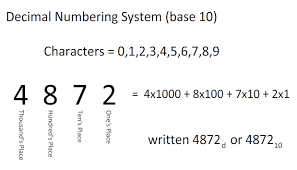You can also convert binary numbers to decimal numbers. To do so, multiply each number by a power of two and then add the products together.

Binary numbers, unlike other numbering systems, are easy to learn and work with us there are no multiplication tables or additional functions associated with them. This explains why binary numbers are often preferred when implementing the circuitry.

But it can be cumbersome to use binary numbers in your everyday mathematics operations and your process will be prone to errors.

It is also possible to convert binary numbers to hexadecimal numbers. In this case, categorize the binary numbers into four groups starting from the right hand and then translate each group of the binary numbers into its equivalent in hexadecimal.

Decimal number system
The decimal numbering system is also commonly referred to as a base-10 positional numeral system or decanary or denary. It is the standard numbering system for representing both integer and non-integer numbers.

Decimal numbers are the extension to non-integer numbers of the Arabic-Hindu numbering system. Denoting numbers in the decimal numbering system is commonly referred to as decimal notation.

Decimal numbers may occasionally be identified for holding decimal separator, for instance, 200.00 or 1.234567. Decimal numbers can be converted to binary number system by using online decimal to binary converter tool. In some instances, decimal may also refer to the numbers that come after the decimal separator, for example, pie 3.14 can be said as pie in approximation to two decimals.

Unlike in the binary numbering system, the decimal numeral system uses digits from 0 to 9.

Decimal numbers use from single to multiple digits to represent higher values of a number, which is linked with a place value or weighting factor. Each value of the place value is associated with power ten.

Decimal numbers can also be expressed as decimal fractions, with the denominator expressed in the power of ten. For example, the number 0.5 can be written as a fraction like 1/2, while 0.8 as 8/10, 13.79 as 1379/100, and so on. From these expressions, we can conclude that a decimal number with n numerals after the decimal separator represents the fraction with a denominator 10n, who’s the numerator is the integer acquired after removing the decimal separator.

The decimal number cannot be represented as an exact for all real numbers. However, they allow for approximation of every real number with any preferred level of accuracy. For example, using the pie in decimal format; 3.14159 approximate the real pie, as it is less than 10-5 off.

Decimal numbering systems are commonly used in science, engineering as well as our everyday life. But in computing, binary, hexadecimal, and octal are widely used instead of decimals.

When writing decimal numbers, the base is usually omitted, as, without it, the number is assumed to be technically a decimal.

According to Tutorialpoint, Each digit in the decimal number system is assigned a weight value. And since decimal numbers are base-ten, numbers, the weight of each digit is in the power of 10. Also, each digit has a position value. To put it simply, the first digit on the rightmost is position zero, and the next digit, moving towards the left, is position one. Moving to the left, the next number is position two, and so on.

The weight of the number in each position is typically the base of the number raised to the power of the position number. However, the value of the number can be calculated by multiplying the value of the number in each position, by the base raised to the power of that particular position and then adding up the products.